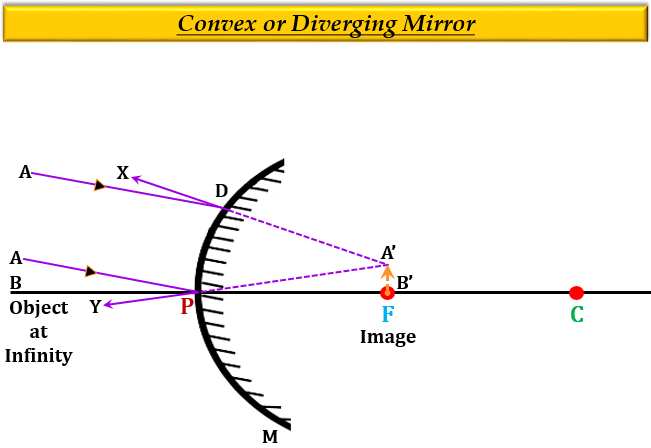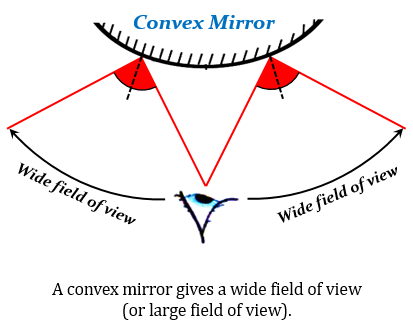# (a) Draw a labelled ray diagram to show the formation of image in a convex-mirror when the object is at infinity. Mark clearly the pole and focus of the mirror in the diagram.(b) State three characteristics of the image formed in this case.(c) Draw diagram to show how a convex mirror can be used to give a large field of view.

(a) Ray Diagram -(b) Three characteristics of the image formed in this case are:

1. virtual (behind the mirror).

2. erect (upright).

3. diminished (smaller than the object).

(c) The following ray diagram shows that a convex mirror can be used to produce a large or wide field of view because it always forms virtual, erect, and diminished images irrespective of the distance between the object and the mirror (other than infinity). Therefore, a convex mirror can produce image of a large number of objects at the same time.Updated on: 10-Oct-2022

90 Views